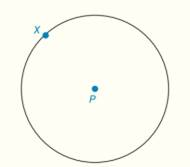Chapter 6.CT, Problem 13CT### Elementary Geometry for College St...

6th Edition
Daniel C. Alexander + 1 other
ISBN: 9781285195698

#### Solutions

Chapter
Section### Elementary Geometry for College St...

6th Edition
Daniel C. Alexander + 1 other
ISBN: 9781285195698
Textbook Problem
12 views

# Construct the tangent line to   ⊙ P at point X .To determine

To construct:

The tangent line to P at point X.

Explanation

Given:

P is a circle with point X on it.

Theorem used:

“The line that is perpendicular to the radius of a circle at its endpoint on the circle is a tangent to the circle”.

Approach:

Let us construct a tangent line to P at point X based on the theorem “The line that is perpendicular to the radius of a circle at its endpoint on the circle is a tangent to the circle”.

Construction:

Step 1:

Consider P with point X.

Step 2:

Draw radius PX¯ and extent it to the form PX.

Step 3:

Using X as a center and any length which is less than PX as radius, draw two arcs which intersects the line segment PX at points Y and Z

### Still sussing out bartleby?

Check out a sample textbook solution.

See a sample solution

#### The Solution to Your Study Problems

Bartleby provides explanations to thousands of textbook problems written by our experts, many with advanced degrees!

Get Started

#### In Exercises 2340, find the indicated limit. 34. limx1x3+12x3+2

Applied Calculus for the Managerial, Life, and Social Sciences: A Brief Approach

#### Evaluate the integral. 13. sin5tcos4tdt

Single Variable Calculus: Early Transcendentals

#### ∫(x − cos x)dx = 1 − sin x + C 1 + sin x + C

Study Guide for Stewart's Single Variable Calculus: Early Transcendentals, 8th